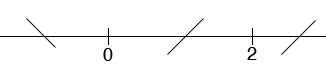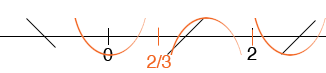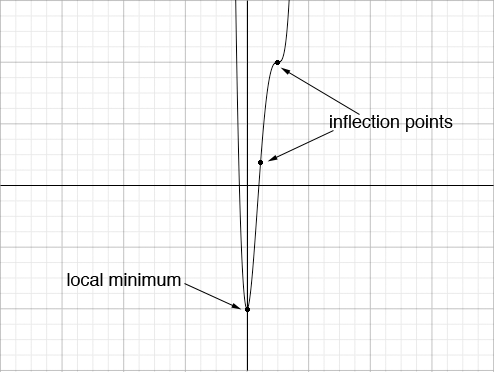SEARCH HOMEMath Central Quandaries & QueriesQuestion from Ahson, a student: Find critical points, determine the monotonicity and concavity and sketch a graph of f(x) with any local maximum, local minimum and inflection points labeled: 1. f(x) = x^4 - x^3 - 3x^2 + 1Ahson,

I'm going to illustrate with f(x) = 3x4 - 16x3 + 24 x2 - 8.

For monotonicity I need the first derivative.

f '(x) = 12 x3 - 48 x2 + 48 x = 12x(x - 2)2

Hence the critical points are at x = 0 and x = 2. These two points separate the line into three parts and I check the monotonicity in each of the three parts.

(-∞, 0): f '(-1) < 0 and hence f(x) is decreasing
(0, 2): f '(1) > 0 and hence f(x) is increasing
(2, ∞): f '(3) > 0 and hence f(x) is increasing

In my rough work I usually mark this on a diagram.For concavity I need the second derivative

f "(x) = 36 x2 - 96 x + 48 = 12(3x - 2)(x - 2)

Hence the curve may change concavity at x = 2/3 and x = 2. Now I check the concavity in the 3 intervals defined by these points.

(-∞, 2/3): f "(0) > 0 and hence f(x) is concave upwards
(2/3, 2): f "(1) < 0 and hence f(x) is concave downwards
(2, ∞): f "(3) > 0 and hence f(x) is concave upwards

I put this information on the same diagram.Now I'm ready to attempt a sketch but I need to plot some points to determine where on the plane the graph lies. I usually plot the critical points if this is feasible.

f(0) = -8
f(2) = 8

Here is my sketch.I hope this helps,
HarleyMath Central is supported by the University of Regina and The Pacific Institute for the Mathematical Sciences.